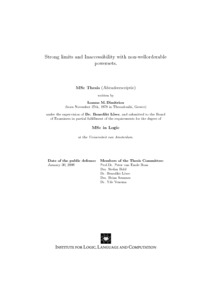# MoL-2006-03: Strong limits and Inaccessibility with non-wellorderable powersets

MoL-2006-03: Dimitriou, Ioanna (2006) Strong limits and Inaccessibility with non-wellorderable powersets. [Report]Preview Text (Full Text) MoL-2006-03.text.pdf Download (508kB) | PreviewText (Abstract) MoL-2006-03.abstract.txt Download (3kB)

## Abstract

This thesis is about set theory without the axiom of choice. The
theory of ordinals and their powersets without the axiom of choice is
not a popular subject in set theoretic practise; in this thesis, we
will shed a little light on some basic questions in this area.

Our basic theory is ZF, unless otherwise stated. In this thesis we
will be interested in the concept of a cardinal being a strong
limit. This concept is one of the basic properties of the ordinary
theory of cardinals and their powersets. It is well studied in the
ZFC context and is typically defined as follows:

\kappa is a strong limit IFF(def) \forall \lambda<\kappa (2^\lambda<\kappa)

where we read "2^\lambda<\kappa" as \some (any) ordinal in bijection
with the powerset of \lambda is smaller than \kappa". In the ZFC
context, this ordinal always exists, if 2^\lambda is not
wellorderable, it may not. As it turns out, this definition is
equivalent in ZFC to four other definitions (where < is replaced by
relations <_s, <_i, <_{s-} <_{i-}) that are more appropriate for an
investigation without the axiom of choice.

We look at this subject from two different points of view, thus this
thesis includes two parts. The first part is looking at the problem
from an axiomatic point of view, i.e., we see what different answers
we can have when we assume different axioms. It starts with the axiom
of choice, the axiom of determinacy, weaker forms of them which are
involved in this study and some generalisations of statements
incompatible with the more famous axioms above. We end this part with
a discussion on several notions of being an inaccessible cardinal,
i.e., a regular strong limit cardinal. These are defined using the
alternative definitions of strong-limitedness we mentioned above. We
also define the notion of being a \beta-inaccessible cardinal that uses
the set of ultrafilters on a cardinal and it is connected with the
axiom of determinacy.

The notion of inaccessibility is connected with a metamathematical
point of view in set theory. It is known that the existence of
inaccessible cardinals is equivalent to ZFC having a set model. By
Goedel's Incompleteness and Completeness Theorems this is actually a
metamathematical proof that these cardinals' existence cannot be
proven in ZFC. From this metamathematical point of view, theorems that
talk about the consistency of a theory motivate us to define a
consistency strength hierarchy between theories that contain ZF. This
is because we conventionally accept ZF as consistent.

We will not go into the details of this hierarchy but we will just state that
a theory T has stronger consistency strength than a theory T' if T can prove
the consistency of the same or more theories than T' can. Therefore the theory
ZFC+"there is an inaccessible cardinal" is stronger than ZFC. All this creates
the natural question of what happens in non-AC environments.

This leads us at the second part of the thesis, where we look at the
problem by constructing generic models by forcing. First we take a
brief look at a model by Blass where all ultrafilters are principal
and where the notion of being a \\beta-strong limit becomes
trivial. Afterwards we will describe the method of taking symmetric
submodels of generic extensions and then we will study in depth the
Feferman-Levy model, a symmetric submodel. This model will answer most
of our questions in this part and this will lead us to attempt a
generalisation of it, hoping this will solve our last question. This
attempt will fail but this failure will make the problem clearer and
might help to lead us in a new way to approach this in the future.

Item Type: Report MoL-2006-03 Master of Logic Thesis (MoL) Series 2006 strong limits, inaccessible cardinals, consistency strength, set theory without the axiom of choice 12 Oct 2016 14:38 12 Oct 2016 14:38 https://eprints.illc.uva.nl/id/eprint/766View Item